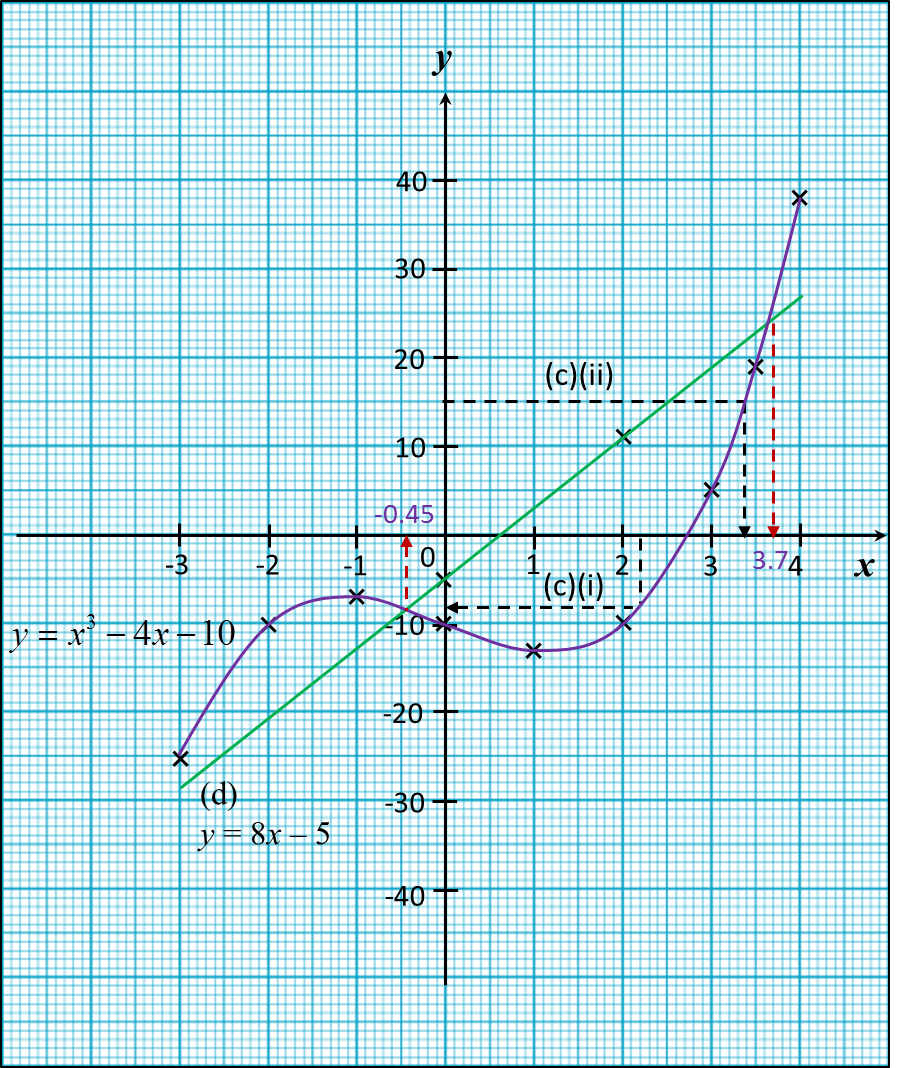# 2.5 Graph of Functions (II), SPM Paper 2 (Long Questions)

Question 7:
(a) The following table shows the corresponding values of x and y for the
equation y= –x3 + 3+ 1.

 x –3 –2 –1 0 1 2 3 3.5 4 y 19 3 r 1 3 –1 s –31.4 –51
Calculate the value of r and s.

(b) For this part of the question, use graph paper. You may use a flexible curve rule.
By using a scale of 2cm to 1 unit on the x-axis and 2cm to 5 units on the y-axis, draw the graph of  y = –x3 + 3x + 1 for –3 ≤ x ≤ 4 and –51 ≤ y ≤ 19.

(c)
From your graph, find
(iThe value of when x = –2.8,
(iiThe value of when y = 30.

(d)
Draw a suitable straight line on your graph to find the values of x which satisfy the equation –x3 + 13x – 9 = 0 for –3 ≤ x ≤ 4 and –51 ≤ y ≤ 19.

Solution:
(a)
y= –x3 + 3+ 1
when x = –1,
= – (–1)3 + 3(–1) + 1
= 1 – 3 + 1 = –1
when x = 3,
= – (3)3 + 3(3) + 1 = –17

(b)

(c)
(iFrom the graph, when x = –2.8, y = 15
(iiFrom the graph, when y = 30, x = 3.5

(d)
y= –x3 + 3x+ 1 —– (1)
x3+ 13x – 9 = 0 —– (2)
y= –x3 + 3+ 1 —– (1)
0 = –x3 + 13x – 9 —— (2) ← (Rearrange (2))
(1)  – (2) : y = –10x + 10

The suitable straight line is y = –10x + 10.

Determine the x-coordinates of the two points of intersection of the curve
y = –x3 + 3+ 1 and the straight line y = –10x10.

 x 0 4 y = –10x + 10 10 –30
From the graph, x= 0.7, 3.25.

Question 8:
(a) Complete the table in the answer space for the equation y = x3 – 4x – 10 by writing down the values of y when x = –1 and x = 3.

(b) For this part of the question, use graph paper. You may use a flexible curve rule.
By using a scale of 2cm to 1 unit on the x-axis and 2cm to 10 units on the y-axis, draw the graph of y = x3 – 4x – 10 for –3 ≤ x ≤ 4 and –25 ≤ y ≤ 38.

(c) From your graph, find
(i) the value of y when x = 2.2,
(ii) the value of x when y = 15.

(d) Draw a suitable straight line on your graph to find the values of x which satisfy the equation x3 – 12x – 5 = 0 for –3 ≤ x ≤ 4 and –25 ≤ y ≤ 38.

 x –3 –2 –1 0 1 2 3 3.5 4 y –25 –10 –10 –13 –10 18.9 38
Solution:
(a)
y = x3 – 4x – 10
when x = –1,
y = (–1)3 – 4(–1) – 10
= –7

when x = 3,
y = (3)3 – 4(3) – 10
= 5

(b)(c)
(i) From the graph, when x = 2.2, y = –8
(ii) From the graph, when y = 15, x = 3.4

(d)
y = x3 – 4x – 10 ----- (1)
0 = x3 – 12x – 5 ----- (2)
(1) – (2) : y = 8x 5

The suitable straight line is y = 8x5. Determine the x-coordinates of the two points of intersection of the curve y = x3 – 4x – 10 and the straight line y = 8x –5.

 x 0 2 y = 8x – 5 –5 –11
From the graph, x = –0.45, 3.7.